• 读论文——A Joint Model for Aspect-Category Sentiment Analysis with Shared Sentiment Prediction ...
2022-04-28 20:34:41

# 第一遍

## 标题以及作者## 摘要

给定一段文本，一些联合模型探查所有的方面词然后预测它们的情感极性。但是它们为每个方面词单独训练参数，因此困扰于某些方面词的数据不足的问题。为了解决这个问题，我们提出了一个新的联合模型，可以共享情感预测层。共享情感预测层在方面类别中传递情感知识，缓解数据不足带来的问题。

## 结论

在这项工作中，我们提出了一种新的联合模型，其中包含一个共享的情感预测层。共享情感预测层在方面类别之间传递情感知识，缓解数据不足带来的问题。在 SemEval-2016 任务 5 的四个数据集上进行的实验证明了我们模型的有效性。未来的工作可以考虑引入额外的组件，以防止共享情感预测层传输特定于方面的情感知识

## 介绍

一个方面类别是一组实体E和属性A对,例如：AMBIENCE#GENERAL

方面类别检测 (ACD)，它检测文本中的方面类别和情感分类 (SC)，它根据检测到的方面类别对情感极性进行分类，并分别执行这两个任务.这种两阶段的方法会导致错误传播，即方面类别检测引起的错误会影响情感分类。

将 ACSA 任务分为两个子任务：方面类别检测 (ACD)，它检测文本中的方面类别和情感分类 (SC)，它根据检测到的方面类别对情感极性进行分类，并分别执行这两个任务.这种两阶段的方法会导致错误传播，即方面类别检测引起的错误会影响情感分类。

不同方面类别的情感表达及其极性是可转移的。例如，在表 1 中，LAPTOP#QUALITY、LAPTOP#PRICE 和 LAPTOP#OPERATION PERFORMANCE 三个方面类别具有相同的情感词“惊讶”和一致的极性。共享情感预测层在方面类别之间传递情感知识，缓解数据不足带来的问题。## 相关工作

现有的Aspect-Category Sentiment Analysis（ACSA）方法可以分为两类：两阶段方法和联合模型。

## 模型### 输入层

X = { x 1 , x 2 , . . . , x n } , x i ∈ R d w X = \{x_1,x_2,...,x_n\},x_i \in \R^{d_w}

h ⃗ i = LSTM ⁡ → ( h ⃗ i − 1 , x i ) h i ← = LSTM ⁡ ← ( h ← i + 1 , x i ) h i = [ h ⃗ i , h ← i ] H = { h 1 , . . . , h n } \begin{gathered} \vec{h}_{i}=\overrightarrow{\operatorname{LSTM}}\left(\vec{h}_{i-1}, x_{i}\right) \\ \overleftarrow{h_{i}}=\overleftarrow{\operatorname{LSTM}}\left(\overleftarrow{h}_{i+1}, x_{i}\right) \\ h_{i}=\left[\vec{h}_{i}, \overleftarrow{h}_{i}\right] \\ H = \{h_1,...,h_n\} \end{gathered}

### Aspect 注意力层

f ( V ) = v = ∑ i = 1 n α i v i u i = tanh ⁡ ( W a v i + b a )  for  i = 1 , 2 , ⋯   , n α i = exp ⁡ ( u i T u w ) Σ j = 1 n exp ⁡ ( u j T u w )  for  i = 1 , 2 , ⋯   , n \begin{gathered} f(V)=v=\sum_{i=1}^{n} \alpha_{i} v_{i} \\ u_{i}=\tanh \left(W_{a} v_{i}+b_{a}\right) \quad \text { for } \quad i=1,2, \cdots, n \\ \alpha_{i}=\frac{\exp \left(u_{i}^{T} u_{w}\right)}{\Sigma_{j=1}^{n} \exp \left(u_{j}^{T} u_{w}\right)} \quad \text { for } \quad i=1,2, \cdots, n \end{gathered}

v A j X = f A j X ( X ) , j = 1 , ⋯   , N v A j H = f A j H ( H ) , j = 1 , ⋯   , N \begin{aligned} v_{A_{j}}^{X} &=f_{A_{j}}^{X}(X), j=1, \cdots, N \\ v_{A_{j}}^{H} &=f_{A_{j}}^{H}(H), j=1, \cdots, N \end{aligned}

计算输入中每个词向量对每个Aspect的注意力分数

### Aspect 类别预测层

v A j = [ v A j X , v A j H ] y ^ A j = σ ( W ^ A j ReLU ⁡ ( W A j v A j + b A j ) + b ^ A j ) σ ( x ) = 1 ( 1 + e − x ) \begin{gathered} v_{A_{j}}=\left[v_{A_{j}}^{X}, v_{A_{j}}^{H}\right] \\ \widehat{y}_{A_{j}}=\sigma\left(\widehat{W}_{A_{j}} \operatorname{ReLU}\left(W_{A_{j}} v_{A_{j}}+b_{A_{j}}\right)+\widehat{b}_{A_{j}}\right) \\ \sigma(x)=\frac{1}{\left(1+e^{-x}\right)} \end{gathered}

### 情感注意力层

v s = g ( X , v q ) β i = exp ⁡ ( x i T v q ) ∑ j = 1 n x j T v q  for  i = 1 , 2 , ⋯   , n v s = ∑ i = 1 n β i x i \begin{gathered} v_{s}=g\left(X, v_{q}\right) \\ \beta_{i}=\frac{\exp \left(x_{i}^{T} v_{q}\right)}{\sum_{j=1}^{n} x_{j}^{T} v_{q}} \quad \text { for } \quad i=1,2, \cdots, n \\ v_{s}=\sum_{i=1}^{n} \beta_{i} x_{i} \end{gathered}

v s j X = g ( X , v A j X ) v s j H = g ( H , v A j H ) \begin{aligned} &v_{s_{j}}^{X}=g\left(X, v_{A_{j}}^{X}\right) \\ &v_{s_{j}}^{H}=g\left(H, v_{A_{j}}^{H}\right) \end{aligned}

### 共享情感预测层

v s j = [ v s j X , v s j H ] y ^ s j = softmax ⁡ ( W ^ s Re ⁡ L U ( W s v s j + b s ) + b ^ s ) softmax ⁡ ( x ) i = exp ⁡ ( x i ) ∑ k = 1 M exp ⁡ ( x k ) \begin{gathered} v_{s_{j}}=\left[v_{s_{j}}^{X}, v_{s_{j}}^{H}\right] \\ \widehat{y}_{s_{j}}=\operatorname{softmax}\left(\widehat{W}_{s} \operatorname{Re} L U\left(W_{s} v_{s_{j}}+b_{s}\right)+\widehat{b}_{s}\right) \\ \operatorname{softmax}(x)_{i}=\frac{\exp \left(x_{i}\right)}{\sum_{k=1}^{M} \exp \left(x_{k}\right)} \end{gathered}

# 第二遍

## 实验

我们对来自 SemEval-2016 任务 5：Aspect-based Sentiment Analysis (Pontiki et al., 2016) 的四个公共数据集进行实验：

CH-CAME-SB1 是一个关于数码相机领域的中文句子级数据集。

CH-PHNS-SB1 是一个关于手机领域的中文句子级数据集。

EN-REST-SB2 是关于餐馆领域的英文评论级数据集。

EN-LAPT-SB2 是关于笔记本电脑领域的英文评论级数据集。

我们以 9:1 的比例将原始训练集随机拆分为训练集、验证集。我们使用四分位数来衡量这些数据集中方面样本大小的分布。详细的统计数据总结在表2中。特别是对于三个数据集CH-CAME-SB1、CH-PHNS-SB1和EN-LAPT-SB2，50%的方面的样本量不超过7。

### 衡量标准

S是预测的集合，G是真实集合。
P = ∣ S ∩ G ∣ ∣ S ∣ R = ∣ S ∩ G ∣ ∣ G ∣ F 1 = 2 ∗ P ∗ R P + R \begin{aligned} &P=\frac{|S \cap G|}{|S|} \\ &R=\frac{|S \cap G|}{|G|} \\ &F_{1}=\frac{2 * P * R}{P+R}\\ \end{aligned}

# 第三遍

## 小结：

1. 本文使用带有共享情感层（由于某些方面词的样本较少，希望可以使用其他方面词样本中的情感词信息）的联合网络结构，实现ACSA任务。
2. 本文使用Glove词向量X，以及使用Bi-LSTM网络结构提取隐变量H，然后分别计算Aspect注意力、Sentiment注意力，经过Aspect注意力层后，可以直接接线性层预测Aspect，综合使用X、H以及Aspect注意力来计算Sentiment注意力层，进行情感预测。
更多相关内容
• 读 《Multi-Instance Multi-Label Learning Networks for Aspect-Category Sentiment Analysis》 这篇论文做的笔记。

## 前提

作者定义：

• bag： sentence；
• instance： word；
• key instance：指某 aspect category 的 key instance，需要是能体现该 aspect category 的 instance。

笔者使用习惯：

• 下划线代表重点；
• 删除线代表不重要（或者是一些套话）。

## Motivation

之前的工作忽略了一个问题，就是事实上预测一个 aspect category 的情感极性需要的是将所有对预测该 category 有用的 word 的情感综合考虑。以前的做法（例如基于 Attention 机制的）是将 sequence encode，然后对 encode 之后的内容进行 attention 处理，这样可以发现，最终预测 aspect 的情感极性用的是 sequence 中词表示的结合（而不是词情感的结合）。

下面我用一个可能没那么恰当的例子（因为这个是自己想的，不是实验测试出来的，旨在说明上一段两种想法）来说明：

“The food is delicious, but drink is just so so.”

人类正常对这句话分析的思维如下：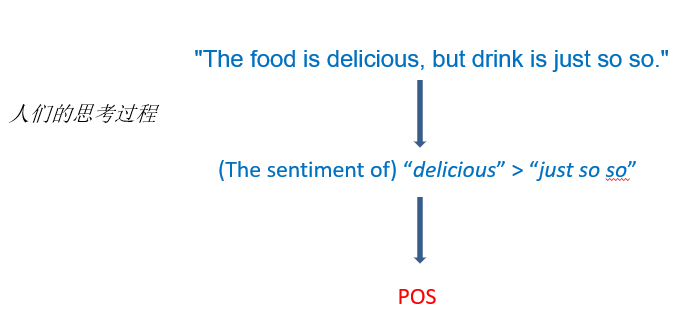这句话中对 “food” 有用的 word 无疑是 “food is delicious”、“drink is just so so”，如果是以前的方法，那么对这个 sequence 做 attention 后，大同小异地就是得到一个针对 “food” 类型的表示，之后基于这个表示得到最后的预测结果；而作者提出的思想是，先得到每个 word 对 “food” 这个类型的情感倾向，之后再用 Attention 来将这些情感倾向组合得到最后的结果。

首先作者提出的这种想法更符合人类的思维，因为 “delicious” 比 “just so so” 在情感表现方面无疑是更强烈的，所以基于此，这句话对 “food” 类型的情感极性预测应该是 “pos”；而以前的做法是将 “delicious”、“just so so” 的表示结合起来，之后再基于此预测，这样会有什么问题呢？就是会使模型的表示能力更强（容易过拟合），可能 model 在之前训练中学到的是拥有复杂描述的修饰词重要性更强，而 “just so so” 的结构显然要更复杂，所以可能给它的重要性权重更大，使得结果预测错误。

上述的这种思想差别可以不太恰当地用下面这个图（例子）来说明：我们看奥特曼打怪兽不指望奥特曼干别的，我们希望他直接锤小怪兽就完事了，而不是打之前还得看看今天天气恶不恶劣，自己昨天晚上睡没睡好。这就像我们希望现在 ACSC 模型所做的，直接聚合每个词的情感作为预测就好了（更强烈的情感主导最后的结果）。而不是把每个词的表示聚合在一起，因为表示中很可能蕴含着情感信息以外的东西，使得考虑的东西复杂了。

PS：在 Jiang et al. (2019) “A challenge dataset and effective models for aspect-based sentiment analysis” 论文中提出的 capsule networks 得到了目前最好的结果。）

## 方法概述

首先作者提出的方法所处理的任务分为两部分：

• Aspect Category Detection (ACD)：推断出 sequence 中提到的 aspect category，这一部分作为辅助任务。
• Aspect Category Sentiment Classification (ACSC)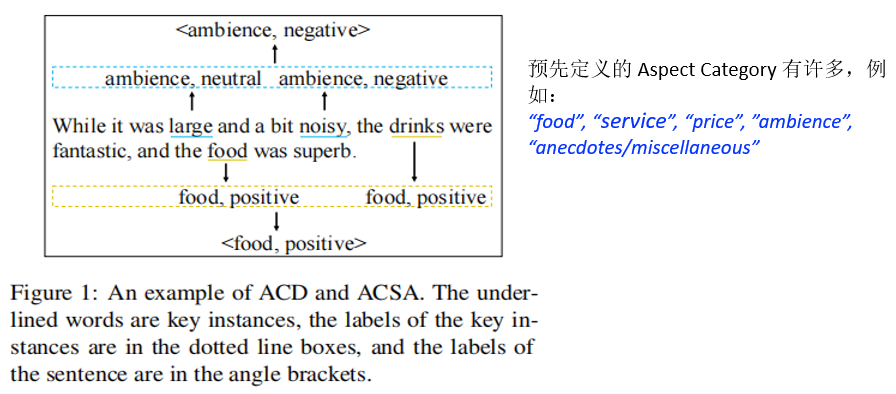接下来说明作者的方法。

作者提出的模型——AC-MIMLLN 符合 Motivation 中所提到的思想，即对 aspect category 情感极性的预测是通过综合考虑各个能表明 aspect category 的词汇的情感的集合。

具体一点来说：

1. 首先该模型将 sequences 视为 bags，其中的 words 视为 instances，能表明 aspect category 的 words 视为该 aspect category 的 key instances。
2. 给一个 bag（sequence）和预先设定好的 aspect categories（同时在该 bag 中提到的）。预测 bag 中所有 instances 的 sentiment（注意：不是 sentiment polarity）。
3. 找到该 bag 中 aspect categories 对应的 key instances。
4. 最后聚合 key instances 的 sentiments 得到 aspect categories 的情感极性。

## 方法详述

### Problem Formulation

首先定义 N N 个预先设定的 aspect categories 为 A = { a 1 , a 2 , … , a N } A = \{a_1,a_2,\dots,a_N\} ，并定义三分类的情感极性为 P = N e g , N e u , P o s P = {Neg,Neu,Pos} 。给定一个句子 S = { w 1 , w 2 , … , w n } S = \{w_1,w_2,\dots,w_n\} 以及该句子中包含的 K K 个 aspect categories， A S = { A 1 S , A 2 S , … , A K S } A^S = \{A^S_1,A^S_2,\dots,A^S_K\} ，其中 A S ⊂ A A^S \subset A

针对这 K K 个 aspect categories，模型需要做的是根据每个句子预测这 K K 个 aspect categories 的情感分布 p = { p 1 , p 2 , … , p K } p = \{p_1,p_2,\dots,p_K\} ，其中 p k = { p k N e g , p k N e u , p k P o s } p_k = \{p_{k_{Neg}},p_{k_{Neu}},p_{k_{Pos}}\}

注意，上面提到的 p p 是一个分布（函数），对于每个 aspect category 是有情感分布，同样，对于每个单词也应该有一个情感分布，最终 aspect category 的情感分布应该由每个词的情感分布计算所得。基于此有如下公式：
p j = f ^ θ w ( w j ) (1) p^j = \hat{f}_{θ_{w}(w_j)} \tag1

p k = g ^ θ S k ( p 1 , p 2 , … , p n ) (2) p_k = \hat{g}^k_{θ_S}(p^1,p^2,\dots,p^n) \tag2

### Multi-Instance Multi-Label Learning Network for ACSA

作者将他提出的 Multi-Instance Multi-Label Learning Network for Aspect-Category sentiment analysis 称作 AC-MIMLLN。

AC-MIMLLN 包含两部分，或者说分为两个分类器，一个是 ACD 分类器，另一个是 ACSA 分类器。整体框架图如 Figure 2 所示。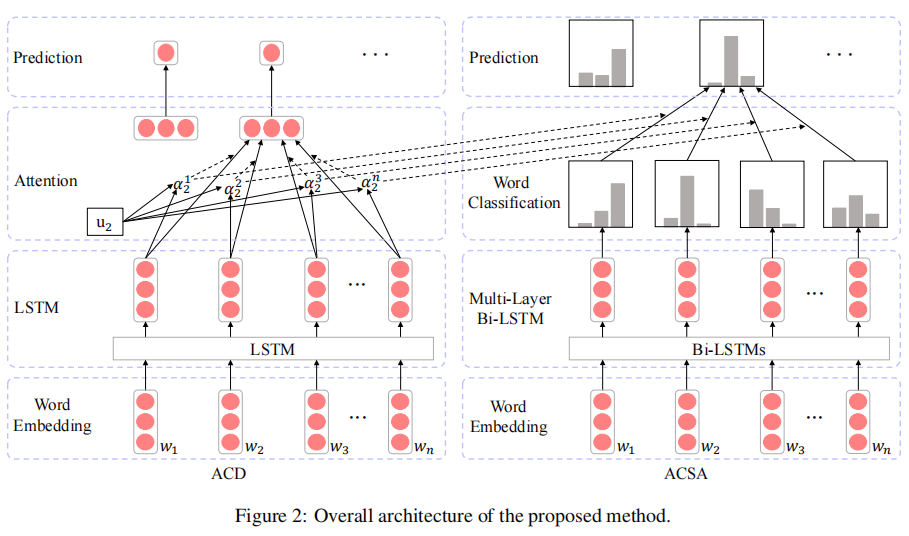图中左边部分为 ACD 分类器，这部分作为辅助任务是要得出每个 word 分别对于几个 aspect categories 的 weights 。得到的 weights 代表着该词成为 aspect categories 的 key instance 的概率；右边部分为 ACSA 分类器，其首先得到每个 word 对于每个 aspect categories 的 sentiment，之后根据 ACD 得到的 weights，将 words 的 sentiments 根据 weights 聚合得到最终各个 aspect categories 的情感预测结果。

需要说明的是，作者设计的 ACD 只是作为一个辅助任务，作者这部分的设计也十分简单，所以效果有待提升。下面详细介绍这个模型：

input：model 的左右两部分的输入是相同的，都是一个 n n 个 word 的 sentence S = { w 1 , w 2 , … , w n } S = \{w_1,w_2,\dots,w_n\}

#### ACD

Embedding layer：将 S = { w 1 , w 2 , … , w n } S = \{w_1,w_2,\dots,w_n\} 转变为对应的一个向量序列 X D = { x 1 D , x 2 D , … , x n D } X^D = \{x_1^D,x_2^D,\dots,x_n^D\}

（PS：这部分大家不说也知道，反正就是得到 words 的 embedding X D X^D

LSTM Layer ：因为 LSTM 本身就可以有选择性的考虑 input，且 Attention 本身也只是为了解决 LSTM 长程依赖与并行化计算而提出的。所以如果 LSTM 本身训练的很有效，那么 Attention 的用处就很有限。而此模型中又必须用到 Attention 得到的 weights，所以为了能够得到好的 weights，所以作者使用了单向 LSTM（以限制其性能）。

该层将 word embedding 作为输入，输出得到隐状态 H = { h 1 , h 2 , … , h n } H = \{h_1,h_2,\dots,h_n\} 。每步 i i 的隐状态计算公式如下：
h i = L S T M ( h i − 1 , x i D ) (3) h_i = LSTM(h_{i-1},x_i^D) \tag3
隐状态的维度依然为 d d

Attention Layer：这一层的目的是计算各个 word 对于每一个 aspect category 的 weight，其中计算某一个 aspect category 的 weights 的计算公式如下：
M j = t a n h ( W j H + b j ) , j = 1 , 2 , . . . , N (4) M_j = tanh(W_jH + b_j ), j = 1, 2, ..., N \tag4

α j = s o f t m a x ( u j T M j ) , j = 1 , 2 , . . . , N (5) α_j = softmax(u_j^TM_j),j = 1, 2, ..., N \tag5

其中 W j ∈ R d × d , b j ∈ R d , u j ∈ R d W_j ∈ R^{d×d},b_j ∈ R^d,u_j ∈ R^d ，均为可学习参数。而 α j ∈ R n α_j ∈ R^n 是求得的注意力权重向量。

计算过程是这样的，代表着所有 words 的隐状态 H H 作为 Attention 的 query 经过线性层后得到 M j ∈ R d × n M_j ∈ R^{d×n} ，之后用其求得注意力权重 α j ∈ R n α_j ∈ R^n

Aspect Category Prediction Layer H H 作为 attention query 的同时也作为 attention 的 value，根据 Attention 得到 words 基于 weights 的最终表示 r j , j = 1 , 2 , … , N r_j,j = 1,2,\dots,N ，计算过程如下：
r j = H α j T , j = 1 , 2 , … , N (6) r_j = Hα_j^T,j = 1,2,\dots,N \tag6
根据得到的 r j , j = 1 , 2 , … , N r_j,j = 1,2,\dots,N 计算第 j j 个 aspect category 的预测结果，计算结果如下：
y ^ j = s i g m o i d ( W j r j + b j ) , j = 1 , 2 , … , N (7) \hat{y}_j = sigmoid(W_jr_j + b_j), j = 1,2,\dots,N \tag7
其中 W j ∈ R d × 1 W_j ∈ R^{d×1} （这里感觉作者笔误了，应该是 W j ∈ R 1 × d W_j ∈ R^{1×d} ） ，并且 b j b_j 是一个标量。

（注意：因为 ACD 是预测该句是否包含某 aspect category，所以该任务对于每个 aspect category 是个二分类问题，所以预测结果 y ^ j , j = 1 , 2 , … , N \hat{y}_j, j = 1,2,\dots,N 是在 0~1 范围内的。）

#### ACSA

Embedding layer：将 S = { w 1 , w 2 , … , w n } S = \{w_1,w_2,\dots,w_n\} 转变为对应的一个向量序列 X C = { x 1 C , x 2 C , … , x n C } X^C = \{x_1^C,x_2^C,\dots,x_n^C\}

Multi-Layer Bi-LSTM：这层也没啥好说的，比较基础的模型组件，多层结构中每一层都以前一层的输出作为输入。形式上，给定第 ( l − 1 ) (l−1) 层的隐状态 H l − 1 = { h 1 l − 1 , h 2 l − 1 ， … ， h n l − 1 } H^{l−1} = \{h_1^{l−1},h_2^{l−1}，\dots，h_n^{l−1}\} ，第 l l 层输出的隐状态为 H l = h 1 l , h 2 l , … , h n l H^l={h^l_1,h^l_2,\dots,h^l_n} 。计算每层中的第 i i 个隐藏状态的公式如下：
h i l → = L S T M → ( h i − 1 l → , h i l − 1 ) (8) \overset{\rightarrow}{h_i^l} = \overset{\rightarrow}{LSTM}(\overset{\rightarrow}{h_{i-1}^l},h_i^{l-1}) \tag8

h i l ← = L S T M ← ( h i + 1 l ← , h i l − 1 ) (9) \overset{\leftarrow}{h_i^l} = \overset{\leftarrow}{LSTM}(\overset{\leftarrow}{h_{i+1}^l},h_i^{l-1}) \tag9

h i l = [ h i l → ; h i l ← ] (10) h_i^l = [\overset{\rightarrow}{h_i^l};\overset{\leftarrow}{h_i^l}] \tag{10}

其中 H 0 = { x 1 C , x 2 C , … , x n C } , h i l → ∈ R d / 2 , h i l ← ∈ R d / 2 , h i ∈ R d H^0 = \{x_1^C,x_2^C,\dots,x_n^C\},\overset{\rightarrow}{h_i^l} ∈ R^{d/2},\overset{\leftarrow}{h_i^l} ∈ R^{d/2},h_i ∈ R^d ，该组件共 L L 层。

Word Sentiment Prediction Layer：将刚刚得到的 h i L h_i^L 用于计算第 i i 个 word 的 sentiment（表示） p i p^i ，计算的方法是利用两次线性转换（即两个全连接层），公式如下：
p i = W 2 R e L U ( W 1 h i L + b 1 ) + b 2 (11) p^i = W^2ReLU(W^1h_i^L + b^1) + b^2 \tag{11}
其中 W 1 ∈ R d × d , W 2 ∈ R d × 3 , b 1 ∈ R d , b 2 ∈ R 3 W^1 ∈ R^{d×d},W^2 ∈ R^{d×3},b^1 ∈ R^d,b^2 ∈ R^3 为可学习参数。

注意：在这几个全连接层之后不设置 softmax 层，因为现在我们要得到的是 sentiment 的表示，如果加入了 softmax 则等于在此预测了每个 word 的情感极性，换句话说会丢失许多情感信息，当然也意味着会增加训练模型的难度。）

Aspect Category Sentiment Prediction Layer：这一层的做法是将刚刚得到的各个 words 的 sentiment representation 按照 ACD 任务得到的 weights 聚合起来，分别得到各个 aspect category 的预测结果。公式如下：
p j = s o f t m a x ( ∑ i = 1 n p i α j i ) (12) p_j = softmax(\sum\limits_{i=1}^np^iα_j^i) \tag{12}
其中 p j ∈ R 3 p_j ∈ R^3 ，并且 α j i α_j^i 代表着的是从 ACD 任务得到的第 i i 个 word 对应于第 j j 个 aspect category 的 weight 。

Loss：对于 ACD 任务和 ACSA 任务，如果单独考虑它们，那么它们有自己单独的 loss。

• 对于 ACD 任务，预测句子中是否隐含了某个 aspect category 的情感是个二分类任务，loss 函数也是常用的最大似然函数：
L A ( θ A ) = − ∑ j = 1 N y j l o g y ^ j + ( 1 − y j ) l o g ( 1 − y ^ j ) (13) L_A(θ_A) = -\sum\limits_{j=1}^Ny_jlog{\hat{y}_j} + (1-y_j)log{(1-\hat{y}_j)} \tag{13}

• 对于 ACSA 任务，只有 sentence 中提及的 K K 个 aspect categoris 才会参与 loss 的计算，公式如下（比较简单）：
L S ( θ S ) = − ∑ j = 1 K ∑ c ∈ P y j c l o g p j c (14) L_S(θ_S) = -\sum\limits_{j=1}^K \sum\limits_{c∈P} y_{j_c}logp{_{j_c}} \tag{14}

因为该模型是个整体，是 ACD 与 ACSA 连接起来的，所以作者将该模型的 loss 结合了两个子任务各自的 loss：
L ( θ ) = L A ( θ A ) + β L S ( θ S ) + λ ∣ ∣ θ ∣ ∣ 2 2 (15) L(θ) = L_A(θ_A) + βL_S(θ_S) + λ||θ||_2^2 \tag{15}

作者通过最小化这个 loss 函数来训练作者的模型。其中 β β 是 loss 的 weight， λ λ L 2 L_2 正则化的参数， θ θ 代表着模型中的（所有）参数。

## 实验分析

### Datasets

Rest14：指的是 SemEval-2014 restaurant review 。

Rest14-hard：按 Xue and Li (2018) 中构建数据集的方法对 Rest14 改造得到的数据集，即在 Rest14 的 test 部分中只保留两个及以上 aspect categories 且情感极性不同的句子。

MAMS-ACSA：因为 Rest14-hard 的 test 部分比较小，所以作者还采用了 Multi-Aspect Multi-Sentiment dataset for Aspect Category Sentiment Analysis（用 MAMS-ACSA 表示）。MAMS-ACSA 提出于 Jiang et al. (2019) ，其中的所有句子每个句子都包含着多个 aspect categories 以及不同的情感极性。

### Comparison Methods

#### Variants of AC-MIMLLN

• AC-MIMLLN – w/o mil：为 ACAC（我认为原文这里笔误，应该是 ACSC，因为我也没听说过 ACAC，也没查到这个任务） 任务生成特定于方面类别的表示。这些表示是基于ACD任务所提供的权重的单词表示的加权和。（作者在文章中这里表述的不是很清楚，我暂时理解其设置这个的意思是，从 ACD 任务中得到的权重，不用于加权每个 instance 的 sentiment，而是加权各个 instance 的隐层表示得到 aspect category-specific representations，之后再做预测。这种做法即 Motivation 提到的以前的做法）。

• AC-MIMLLN-Affine用仿射隐藏层替换AC-MIMLLN中的LSTM，用于评估AC-MIMLLN中注意力的有效性 (Wiegreffe and Pinter, 2019)

• AC-MIMLLN-BERT：将 embedding layer 和 multi-layer Bi-LSTM 替换成 uncased basic pre-trained BERT。替换之后作者为了有效地利用 BERT，所以使用了 CapsNet-BERT 中 input 的形式—— “[CLS] sentence [SEP] aspect category [SEP]”。

可能有人会和我有同样的疑惑：即作者提出的模型的 input 只有 sentence S = { w 1 , w 2 , … , w n } S = \{w_1,w_2,\dots,w_n\} ，而如果用 “[CLS] sentence [SEP] aspect category [SEP]” 作为输入的话则会多出 “[SEP] aspect category [SEP]” 部分，这多出来的部分有用么？

询问了师兄，他说按这种形式送入 BERT 得到的表示确实会更好，至于多出来的部分，师兄说这部分得到的表示不用就行了，无所谓的。

### Experimental Results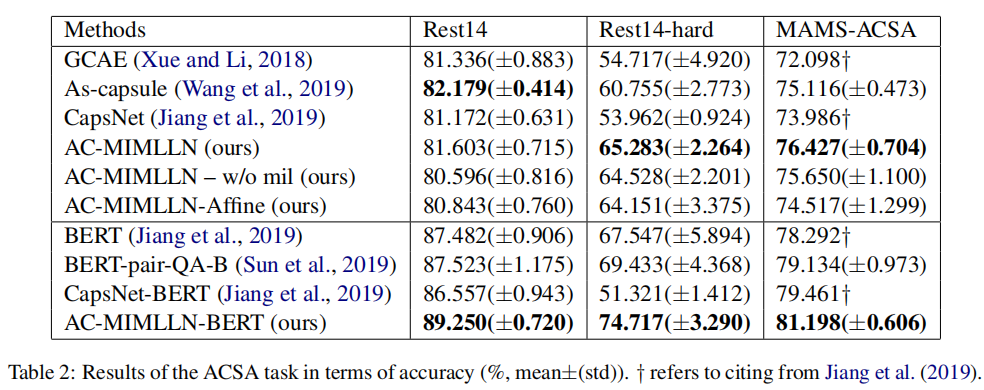实验结果如 Table 2 所示：

1. AC-MIMLLN 在 Rest14-hard 数据集和 MAMS-ACSA 数据集上的性能优于所有none-BERT baselines，这表明 AC-MIMLLN 能够更好地检测一个句子中针对不同方面类别的多个不同的情绪极性。
2. AC-MIMLLN 与 AC-MIMLLN – w/o mil 相比，在 Rest14-hard 数据集上准确率高 1.0%，在 Rest14-hard 硬数据集上准确率高 0.8%，在 MAMS-ACSA 数据集上准确率高 0.8%，这表明，Multiple Instance Learning (MIL) 框架更适合于 ACSA 任务。
3. AC-MIMLLN-BERT 在所有的三个数据集上都超过了所有基于 BERT 的模型，这表明AC-MIMLLN可以通过使用更强大的 ACSA 句子编码器来实现更好的性能。
4. AC-MIMLLN在 Rest14 数据集上的性能低于 As-capsule，主要原因是 AC-MIMLLN 在处理 aspect category misc（anecdotes/miscellaneous 的缩写）时效果比较差。（见 Table 3 和 Figure 4(f)）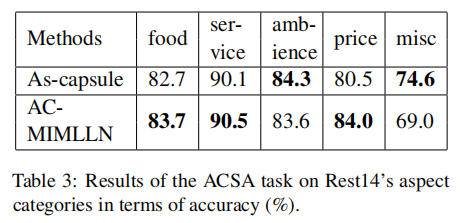在本节中，作者探索了 AC-MIMLLN 在 ACSA 任务的不同多任务设置中的性能。基于此，作者做出了 4 个设置，分别是：“single-pipeline”，“single-joint”，“multi-pipeline” 和 “multi-joint” 。其中：

• single：模型每次只预测一种 aspect category 的情感。
• multi：模型每次预测所有 aspect categories 的情感。
• pipeline：分开训练 ACD 模型和 ACSA 模型，训练好一个之后再训练另一个。
• joint：将 ACD 模型和 ACSA 模型连接起来一起训练。

（PS：AC-MIMLLN 属于 multi-joint。）

针对这部分的实验结果展示在 Table 5 中，如下：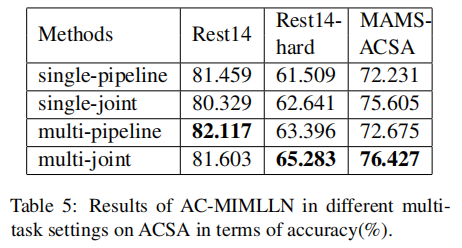首先可以看到 “multi” 类型的方法比 “single” 类型的方法的表现要好。其中 “single” 结果较差的原因我认为是其在计算 loss 时退化成了 3 分类，而 multi 是 3^K 分类，计算效果上存在差距。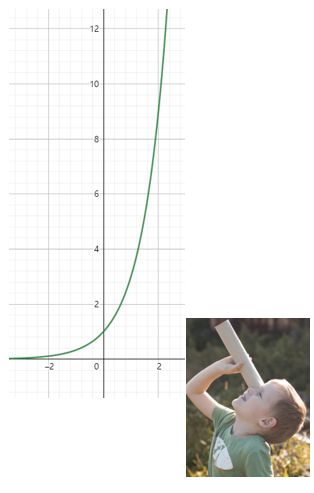其次，“joint” 的效果除了在 Rest14 上的效果比 “pipeline” 差一点以外，在其余两个数据集上表现的都更好。

• 在 Rest14-hard 和 MAMS-ACSA 数据集 “joint” 效果更好的原因就是 “Multi-Task Learning” 的优势，这种合起来效果比单独分开效果好的原因可类比下面这张图：一对筷子合起来设计才好用，如果分开设计，即使每根筷子各自都很好但是合起来反而不好用。

• 在 Rest14 数据集上的结果可以解释为 “joint” 的模型能力更强，而 Rest14 数据集很简单，所以其在 Rest 14 上很容易发生 overfitting。

### Quality Analysis

这一部分通过一些典型的例子介绍作者提出的模型优势以及缺陷，同时这部分评估了该模型处理下面提到的两个任务中的性能，这两个任务分别是 “detecting the key instances (KID)” 任务和给定 aspect category 情况下的 “classifying the sentiments of the given key instances (KISC)” 任务。

下面 Figure 4 中为做的案例实验的结果：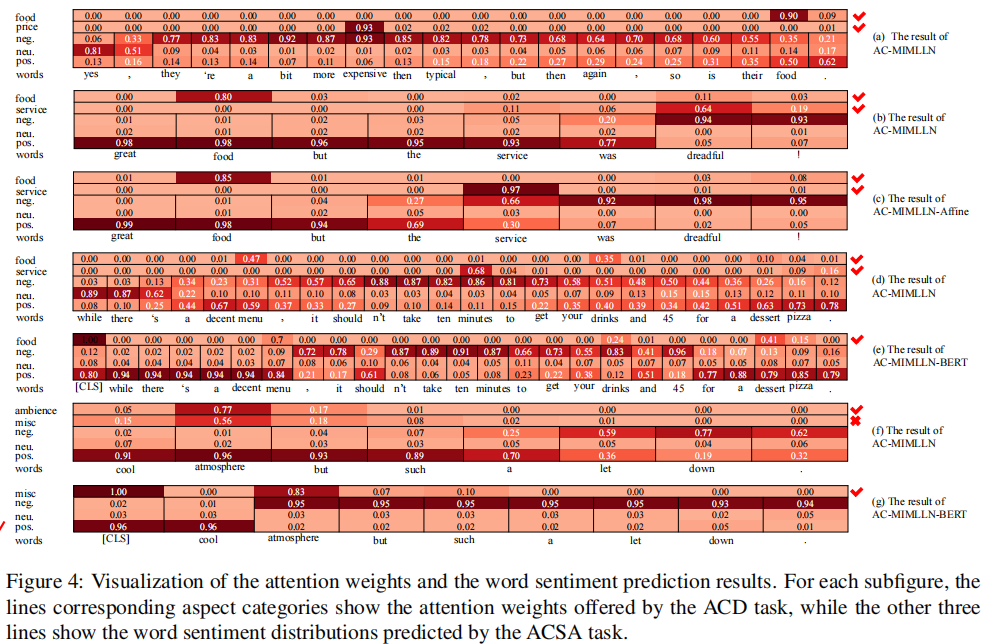case study：Figure 4 (a) 中显示了模型正确找到了给定的 words 中 “food” 和 “price” 的 key instances 分别为 “food：0.90” 和 “expensive：0.93”，并给这几个 key instances 分配了基本正确的情感。所以这个样例解释了作者的模型 ACD 对 ACSA 的帮助作用。

在 Figure 4 (b)、© 显示，ACMIMLLN 和 AC-MIMLLN-Affine 都可以正确地预测方面类别 foodservice 的 sentiment。其中 AC-MIMLLN-Affine 准确地找到了 service 的 key instance “service”，而 AC-MIMLLN 为文本片段 “service was dreadful!” 中的所有单词分配权重。这是因为，ACMIMLLN 中的基于 LSTM 的ACD模型可以根据上下文选择 ACD 和 ACSA 均有用的词，从而获得更好的性能（见 Table 2）。这也可以解释为什么 AC-MIMLLN 在检测给定 aspect category 的 key instances 方面比 AC-MIMLLN-Affine 的性能差（见 Table 4）。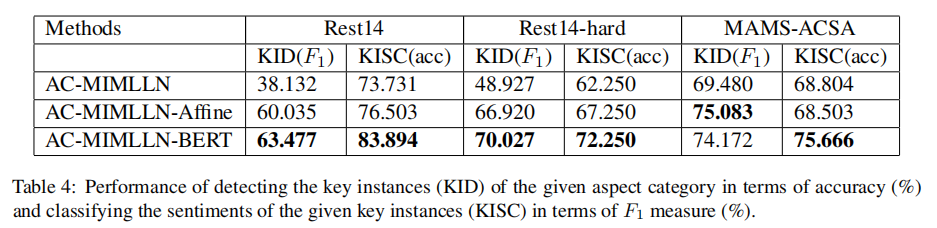Error Analysis在 Figure 4 (f) 中，AC-MIMLLN 错误地预测了 aspect category misc 的 sentiment，因为其找错了相应的 key instances。 这部分作者也没给出什么解释，图中还有一些其他的例子，在此不一一表述了。

## 不懂

• ACAC 是什么任务？

## 改进

• 在预测情感极性时，聚合的是每个词的情感，这有些奇怪。我仍以 “The food is delicious, but drink is just so so.” 说明，显然 “just so so” 作为一个整体才能表示其所蕴含的情感，但是如果将其拆成一个个 word 分别计算其情感，得到的结果可能没什么太大的意义。

## References

1. Wei Xue and Tao Li. 2018. Aspect based sentiment analysis with gated convolutional networks. In Proceedings of the 56th Annual Meeting of the Association for Computational Linguistics (Volume 1: LongPapers), pages 2514–2523.
2. Qingnan Jiang, Lei Chen, Ruifeng Xu, Xiang Ao, and Min Yang. 2019. A challenge dataset and effective models for aspect-based sentiment analysis. In Proceedings of the 2019 Conference on Empirical Methods in Natural Language Processing and the 9th International Joint Conference on Natural Language Processing (EMNLP-IJCNLP), pages 6281–6286.
3. Yequan Wang, Aixin Sun, Minlie Huang, and Xiaoyan Zhu. 2019. Aspect-level sentiment analysis using ascapsules. In The World Wide Web Conference, pages 2033–2044.
4. Chi Sun, Luyao Huang, and Xipeng Qiu. 2019. Utilizing bert for aspect-based sentiment analysis via constructing auxiliary sentence. In Proceedings of the 2019 Conference of the North American Chapter of the Association for Computational Linguistics: Human Language Technologies, Volume 1 (Long and Short Papers), pages 380–385.
5. Sarah Wiegreffe and Yuval Pinter. 2019. Attention is not not explanation. In Proceedings of the 2019 Conference on Empirical Methods in Natural Language Processing and the 9th International Joint Conference on Natural Language Processing (EMNLPIJCNLP), pages 11–20.
展开全文自然语言处理 神经网络
• ACSA主要集中于方面词类别检测Aspect category detection）和方面词情感极性识别（Aspect category polarity identify），以往的方法大多采用流水线的形式，分别实现两个子任务。本文采用一种端到端的联合学习方式...

# 第一遍

## 标题以及作者Joint Learning for Aspect Category Detection and Sentiment Analysis in Chinese Reviews

## 摘要

ACSA主要集中于方面词类别检测（Aspect category detection）和方面词情感极性识别（Aspect category polarity identify），以往的方法大多采用流水线的形式，分别实现两个子任务。本文采用一种端到端的联合学习方式完成ACSA，实验表明本文方法比基线模型更加简单有效。

## 结论

1. 本文使用CNN结构学习字符级表示，并将其与预训练词向量结合，作为最终的词级别表示。
2. 使用highway网络修改输入向量，使其更利于网络的训练。（残差结构？
3. 在词表示基础上，使用另一种CNN结构学习句子表示，使用平均池化和最大池化来获取最后的句子表示，该句子表示最终输入到表示不同类别的不同分类器中。
4. 展望：利用句法树与词法特征捕捉方面词和情感词之间的关系，并与神经网络融合，进一步提高模型的性能。

## 1 介绍

1. 当使用流水线策略时，模型的错误从上游任务转移到下游任务，导致错误的累加。

本文主要贡献：

1. 本文的联合学习模型使用一个共享的编码层和一个独立层解决ACSA任务。编码层用来学习识别不同方面类别共享的句子表示，独立层用来修正不同方面类别的共享句子表示。
2. 本文模型在一个中文评论数据集上进行对比试验，本文模型优于使用复杂结构和大规模参数的基线模型，显示了本文模型的简单性有效性

## 2 相关工作

ACSA之前的工作主要可以分为两类：1. 基于特征的方法，通常使用手工特征，例如：n-gram和字典特征，然后为每个方面类别训练一系列one-vs-all分类器。 2. 基于神经网络的方法

# 第二遍

## 3 我们的模型

### 3.1 模型架构下方的虚线框中参数是共享的，为了识别不同的方面类别而生成句子表示；上方的虚线框中参数是各自独立的，根据生成的相同的句子表示进行方面类别的情感极性分类。

### 3.2 字符级别表示和词级别表示

处理流程：

1. 输入评论句子 S = w 1 , w 2 , . . . , w n S = {w_1, w_2, ...,w_n} ，n表示句子单词个数
2. 每个词可以被表达为一个稠密向量，但是有超过24%的词语OOV，其中一些词语是情感词；因此，本文采用CNN去学习字符级别表示来消除这种影响
3. 模型框架如下：框架详解：

1. 本文将一个句子序列分解成字符序列，例如：“这个车性价比很高”，被划分为5个字符序列，字符序列是随机初始化的，字符序列最大长度为10，每个字符用向量 c i c_i 表示。

2. 卷积操作是在不同的字符向量 c i c_i 间进行，卷积公式如下：
z i = f ( W ⋅ c i : c i + k + b ) z_{i}=f\left(W \cdot c_{i}: c_{i+k}+b\right)

3. 最大池化操作，在特征维度降维，生成一个字符序列向量。

### 3.3 Highway Network 和 句子级别表示

Note：Highway network表示网络中的信息没有任何阻碍的经过网络中的所有层。

highway block：
x dense  = tanh ⁡ ( W 1 x + b 1 ) x gate  = sigmoid ⁡ ( W 2 x + b 2 ) x highway  = x dense  ∗ x gate  + ( 1 − x gate  ) ∗ x \begin{gathered} x_{\text {dense }}=\tanh \left(W_{1} x+b_{1}\right) \\ x_{\text {gate }}=\operatorname{sigmoid}\left(W_{2} x+b_{2}\right) \\ x_{\text {highway }}=x_{\text {dense }} * x_{\text {gate }}+\left(1-x_{\text {gate }}\right) * x \end{gathered}
在highway block顶部使用另一个CNN学习句子表示（本文认为方面词和观点词距离较近，使用卷积操作更加合适），卷积操作之后分别最大池化和平均池化，并将最后结果concatenate得到最终的句子级别表示 S S

### 3.4 联合学习

将得到的句子表示 S S 分别送入不同的分类器中
S ∗ = ( W i s S + b i s ) q i ( x ) = softmax ⁡ ( W i S ∗ S ∗ + b i S ∗ ) \begin{gathered} S^{*}=\left(W_{i}^{s} S+b_{i}^{s}\right) \\ q_{i}(x)=\operatorname{softmax}\left(W_{i}^{S^{*}} S^{*}+b_{i}^{S^{*}}\right) \end{gathered}
L o s s i Loss_i 表示每个子任务的损失
Loss ⁡ i = − ∑ j = 1 N p i ( x ) log ⁡ ( q i ( x ) ) \operatorname{Loss}_{i}=-\sum_{j=1}^{N} p_{i}(x) \log \left(q_{i}(x)\right)
总损失根据各损失加权得到，权值根据训练过程发生变化
Loss ⁡ = ∑ i = 1 n α i L o s s i \operatorname{Loss}=\sum_{i=1}^{n} \alpha_iLoss_i

## 4 实验结果和分析

### 4.1 数据集

2018 CCF大数据与计算智能大赛

### 4.2 实验设置### 4.3 评估指标

定义任务对：某个方面类别的ACD成功+ACSA成功

TP：完成评论中所有任务对

FP：任意任务对失败

FN：预测任务对数量少了

FP：预测任务对数量多了
P = T p T p + F p , R = T p T p + F n , F 1 = 2 P R P + R P=\frac{T_{p}}{T_{p}+F_{p}}, R=\frac{T_{p}}{T_{p}+F_{n}}, F 1=\frac{2 P R}{P+R}

### 4.5 实验结果和分析

#### 4.5.1 与基线模型结果比较#### 4.5.2 字符级别表示有效性

去除CNN-字符表示层，进行对比实验，实验表示加入字符表示结果在开发集上提升1.76%

#### 4.5.3 词嵌入影响分析# 第三遍

## 小结：

1. 本文2019年的论文，本文首次提出了ACSA任务的联合学习模型，不仅使用了预训练模型生成的词向量，同时为了克服OOV问题，也使用了字符序列表示，融合词向量和字符序列表示生成句子表示，然后使用多任务学习的方法，根据相同的句子表示进行方面词类别抽取和方面词情感极性判断。
2. 本文主要使用的网络结构有：CNN、最大池化、平均池化、Highway Network、以及一些正则化操作。
展开全文深度学习 神经网络
• Beta Distribution Guided Aspect-aware Graph for Aspect Category Sentiment Analysis with Affective Knowledge 1. Abstract 本文从新的角度研究了方面类别情绪分析(ACSA)任务，探索一个基于外部知识的Beta分布...

## Beta Distribution Guided Aspect-aware Graph for Aspect Category Sentiment Analysis with Affective Knowledge

### 1. Abstract

本文从新的角度研究了方面类别情绪分析(ACSA)任务，探索一个基于外部知识的Beta分布引导的方面感知图构造。也就是说，本文不再纠缠如何费力地搜索情绪线索的方面，而是如何基于公共知识库更好地找到单词高度情感相关的上下文方面并确定他们的重要性，以便学习方面相关的上下文情感依赖与ACSA这些词。具体地说，首先将每个方面视为一个支点，从外部情感常识知识中衍生出与该方面高度相关的方面感知词汇。然后，使用Beta分布来导出方面感知权重，每个方面感知权重反映了对方面的重要性。然后，将方面感知词作为粗粒度方面的替代品来构建图，以利用ACSA中特殊化的上下文情绪依赖关系。在6个基准数据集上的实验表明，我们的方法显著优于最先进的基线方法。

### 2. Introduction

尽管现有的方法取得了很好的进展，但它们通常都纠结于如何从上下文中搜索粗粒度方面的情感线索。然而，从纯粹基于内隐方面的语境中理解面向方面的情感词汇是一项艰巨的任务。这主要是由于1)方面类别通常不会在上下文中表现出来，2)多个方面和在同一上下文中可能会提到的情感极性。相反，可以利用句子中明确出现的与方面相关的词来建模该方面的上下文情感信息。如图1所示，在句子中有一些与方面相关的词（例如，“地方”、“昂贵”、“披萨”和“美味”），可以明确地利用情感依赖与这些词来识别情感极性的方面。在此基础上，本文从一个新的角度来研究ACSA任务，提出为相应方面的上下文构建方面感知图。更具体地说，将不同的方面视为不同的轴，然后从外部知识中搜索与方面相关的词，称为方面感知词，作为粗粒度方面的替代品，为特定方面构造上下文图。此外，受到许多以前基于图的方法的启发，图中边的权值对于图信息的聚合非常重要。此外，根据实证研究还发现，方面意识词对方面的贡献明显不同。例如，对于方面实体“餐厅”来说，方面感知词汇“地方”比“披萨”更重要。随后，该想法的主要挑战发展为如何确定具有感知方面的词对相应方面的重要性，可以利用图中边的权重来学习该方面的情感线索。

从知识库的角度来看，一个单词可以通过各种路径连接或不连接一个方面，成功的连接概率（对应于图中一条边的权重）可以自然地看作是二项分布。因此，本文通过Beta分布，基于外部知识的先验知识（路径和连接信息）建模所有成功连接可能性的概率来检验边的权重，这是二项分布的共轭先验分布。通过这种方式，可以调查所有连接到方面的方面感知词的概率，从而确定方面感知词的最佳置信概率（权重），称为方面感知权重。随后基于与其权重配对的方面感知词，为每个上下文构建方面感知图。

#### 3. Contribution

在此基础上，提出了一种方面感知图卷积网络(AAGCN)结构，以绘制与ACSA方面的上下文情感依赖关系。主要贡献总结如下：

1. ACSA任务从一个新颖的角度学习如何找到与方面高度相关的相关词汇，并推断其对方面的重要性，从而用这些词汇构建一个图，学习ACSA中的上下文情绪特征。
2. 采用了一种使用Beta分布来建模方面感知词的所有重要概率的新场景，以获得方面感知的权值来构建知识增强的方面感知图。
3. 提出了一种方面感知的图卷积网络，将上下文情绪依赖性绘制到情绪检测的方面，并取得了最先进的性能。

#### 4. Method本节详细描述作者所提出的点图卷积网络(AAGCN)。如图2所示，模型由三个主要部分组成：1)Aspect-aware words derivation，它为从外部知识的不同方面生成一系列不同的情感词汇。2) Aspect-aware graphs construction，它基于方面感知词构建上下文的方面感知图。3)Aspect-aware sentiment learning，它基于方面感知图和上下文表示，提取与方面相关的情绪依赖关系。

给定一个句子 s 由 n 个 单 词 s = { w 1 , w 2 , . . . , w n } s由n个单词s=\{w_1,w_2,...,w_n\} 和相应的方面a组成，这可能不会出现在 ( { w i } ∣ i = 1 , 2 , . . . , n ) (\{w_i\}|i=1,2,...,n) 中。方面类别情绪分析的目标是从上下文中检测出该方面的情绪极性（即积极、消极或中性）。在这里，每个方面可以包括实体E和属性A(即E#A)或仅包括实体E。

##### 4.2 Aspect-aware Words Derivation

为句子中没有出现的方面构建上下文情绪依赖图，本文将一个方面视为支点，并通过在一定数量的跳数中搜索与外部情感知识高度相关的词来获得具有方面感知的词。具体来说，如果单词包含与方面的直接关系，那么这些单词就是1跳方面的单词。相应地，如果单词包含与1跳方面感知单词的关系，那么这些单词就是2跳方面感知单词，等等。此外，如果一个方面由E#A组成，则分别为实体E和属性A寻找方面感知词，因为E和A的角色在情感检测中通常有所不同。

在这种情况下，从直觉上来说，主要的挑战是确定每个有方面意识的词对这个方面的情感重要性。总的来说，跳数是大致重要的影响。然而，如图3(a)和(b)所示，带有2跳的黄色点只包含一个唯一的链接，比同时连接到许多其他不相关单词的绿色点更重要。对于每个单词，它要么连接到κ−跳中的方面，要么不连接，每个感知方面的单词都有一个潜在的Beta分布，揭示了该方面的相关度的分布。因此，基于外部知识学习到的先验知识，本文采用Beta分布，它通常被用来建模一个实验的所有成功概率，以得出每个方面感知词的重要性 ρ ( w i ) ρ(w_i)

ρ ( w i ) = 1 − C D F ( f ( μ ; α , β ) ) ρ(w_i)=1-CDF(f(\mu;\alpha,\beta))

μ i = γ 1 C i α − C i s C i α + γ 2 N κ N \mu_i=\gamma_1 \frac{C_i^\alpha-C_i^s}{C_i^\alpha}+\gamma_2 \frac{N^κ}{N}

其中， C D F ( f ( μ ; α , β ) ) CDF(f(\mu;\alpha,\beta)) 表示 f ( μ ; α , β ) f(\mu;\alpha,\beta) 的累积分布。这里 µ i µ_i 表示方面感知词 w i w_i 的不相关概率， C i a 是 知 识 中 w i C_i^a是知识中w_i 的邻居计数， C i s 是 C_i^s是 方面软件邻居的计数。 N κ 为 κ N^κ为κ −跳方面感知词的词汇量大小， N 为 整 个 语 料 库 的 词 汇 量 大 小 。 γ 1 和 γ 2 N为整个语料库的词汇量大小。γ_1和γ_2 是控制不相关邻居和跳数影响的系数。也就是说，在推导方面感知权值时，作者考虑了不相关的邻居和跳数的影响。如图4中所示的例子，对于“食物”，单词“美味”比“红色”更重要，尽管它的跳数更大。 f ( μ ; α , β ) f(\mu;\alpha,\beta) 表示所有重要概率 θ 的 B e t a θ的Beta 分布，定义为：

f ( μ ; α , β ) ≜ θ α − 1 ( 1 − θ ) β − 1 B ( α , β ) f(\mu;\alpha,\beta) \triangleq \frac{\theta^{\alpha-1}(1-\theta)^{\beta-1}}{B(\alpha, \beta)}

B ( α , β ) ≜ ∫ 0 1 θ α − 1 ( 1 − θ ) β − 1 d θ B(\alpha,\beta)\triangleq \int_0^1 \theta^{\alpha-1}(1-\theta)^{\beta-1}d\theta

其中， B B 是用于归一化的Beta函数。这里 α 和 β 表 示 B e t a 分 布 的 B e t a 分 α和β表示Beta分布的Beta分 布参数：

在此基础上，可以为每个方面感知词获得一个像样的方面感知权重。此外，本文将方面本身和每个无关单词的方面感知权重分别设置为1和0。

##### 4.3 Aspect-aware Graph Construction

本节描述一个关于这些方面的构造上下文依赖图的新解决方案，假设这些方面不会出现在句子中。基于方面感知词及其方面感知权重，作者计算了方面标注图中每个词对的边缘权重如下：

A i , j = ρ ( w i ) + ρ ( w j ) A_{i,j}=ρ(w_i)+ρ(w_j)

受到许多先前基于图的研究的启发，作者还使用句子的依赖树来更好地捕捉句法关系。也就是说，如果 w i 和 w j w_i和w_j 在句子的依赖树中包含依赖关系，则给 A i , j A_{i,j} 的边权值加1。然后构造无向图来丰富情感和依赖信息： A i , j = A j , i A_{i,j}=A_{j,i} ，并为每个单词设置一个自循环： A i , i = 1 A_{i,i}=1

##### 4.4 Aspect-aware Sentiment Learning

对于每个句子，首先从嵌入查找表 V ∈ R m × N V∈R^{m×N} 中检索到句子中每个单词的嵌入情况。因此，对于一个有 n n 个单词的句子，可以得到相应的嵌入矩阵 X = [ x 1 , x 2 , . . . , x n ] X=[x_1,x_2,...,x_n] ，其中 x i ∈ R m 是 w i x_i∈R^m是w_i 的单词嵌入，在训练过程中进行了微调。 M M 是嵌入的维数。然后，将嵌入矩阵 X X 作为输入输入到双向LSTM(Bi-LSTM)层中，推导出句子的隐藏上下文表示：

H = { h 1 , h 2 , . . . , h n } = B i − L S T M ( X ) H=\{h_1,h_2,...,h_n\}=Bi-LSTM(X)

其中 h t ∈ R 2 m h_t∈R^{2m} 表示由Bi-LSTM层导出的时间步长 t t 的隐藏表示。

在此基础上，将句子的方面感知图和隐藏的上下文表示法 H H 输入到方面感知的GCN中，以绘制出该方面对上下文情绪的依赖关系。对于由E#A组成的方面，使用了一个新的交互式GCN块来捕获实体和属性之间的潜在交互。第1个GCN块中的每个节点根据其在实体和属性图邻接矩阵中的隐藏表示进行更新，过程定义为：

f i l = R e L U ( A ~ i e g i l − 1 W e l + b e l ) f_i^l=ReLU(\tilde A_i^eg_i^{l-1}W_e^l+b_e^l) （8）

g i l = R e L U ( A ~ i a f i l W a l + b a l ) g_i^l=ReLU(\tilde A_i^af_i^{l}W_a^l+b_a^l)

其中， g l − 1 g^{l−1} 是从前面的GCN块演化而来的隐藏表示。 A ~ \tilde A 是一个归一化的对称邻接矩阵：

A ~ i = A i / ( E i + 1 ) \tilde A_i=A_i/(E_i+1)

其中 E i = ∑ j = 1 n A i , j 为 A i E_i=\sum^n_{j=1}A_{i,j}为A_i 的度。这里，第一个GCN块的原始输入节点从Bi-LSTM层学习的隐藏表示中检索，即 g 0 = H g_0=H 。此外，对于仅由E组成的方面，方面感知GCN使用等式 (8)进行更新。受(Zhangetal.，2019)的启发，本文采用了一种基于检索的注意机制来捕捉与重要的上下文方面相关的情绪线索：

ζ = ∑ i = 1 n h t ⊤ g i L , η t = exp ⁡ ( ζ t ) ∑ i = 1 n exp ⁡ ( ζ i ) \zeta=\sum_{i=1}^n h_t^\top g_i^L, \eta_t=\frac{\exp(\zeta_t)}{\sum_{i=1^n \exp(\zeta_i)}}

因此，方面感知情绪特征的最终表现如下：

y = softmax ( W o r + b o ) y=\text{softmax}(W_or+b_o)

r = ∑ t = 1 n η t h t r=\sum_{t=1}^n \eta_th_t

##### 4.5 Model Training

本文的任务的目标是通过最小化预测分布和地面真实分布之间的交叉熵损失来训练分类器：

L = − ∑ i = 1 S ∑ j = 1 C y ^ i j ⋅ log ⁡ ( y i j ) + λ ∣ ∣ Θ ∣ ∣ 2 \mathcal{L}=-\sum_{i=1}^S\sum_{j=1}^C \hat y_i^j·\log(y_i^j)+\lambda||\Theta||_2

其中， S 为 训 练 规 模 ， C 为 类 别 数 。 y ^ 是 情 S为训练规模，C为类别数。\hat y是情 感的真实分布。 λ λ 是L2正则化项的权重。 Θ Θ 表示所有可训练的参数。

#### 5. Experiments

##### 5.1 Dataset and Experiment Setting数据集的统计数据如表1所示。对于没有开发集的数据集，我们选择10%的训练集作为验证集，以调整超参数。

对于非bert模型，使用GloVe将每个单词初始化为300维的嵌入。隐藏的向量维数是300。GCN块为2。 γ 1 和 γ 2 γ_1和γ_2 的系数分别为0.4和0.6， λ λ 为0.00001，是初步研究中的最优超参数。使用Adam作为优化器，学习率为0.001，小批量为16。在嵌入层后应用了一个0.3的dropout。对于基于bert的模型，使用预先训练的未简化的bert-base，768维嵌入4，学习率为0.00002。SeniceNet包含了词汇之间的情感常识关系，这在本研究中被用来派生方面意识词汇。将最大跳数设置为5。报告的结果是10次运行的平均得分，以获得统计上稳定的结果。

##### 5.2 Comparison Models##### 5.3 Experiment Results

如表2所示，在6个数据集上的实验结果表明，本文提出的模型在非bert、基于bert和基于bert的模型以及E#A和E方面的性能始终优于比较模型。这验证了所提出的模型在ACSA中的有效性。

与没有使用分布来推导方面感知权重的模型相比，在任何分布中，性能都得到了总体提高。这意味着，探索分布来建模单词和相应方面之间的成功连接概率，更能适应从外部知识中获得更有价值的方面感知权重。此外，由不同分布产生的结果表明，本文的AAGCN，它探索Beta分布来确定方面感知的权重，明显优于几个相关分布。这意味着部署Beta分布来基于从外部知识中学习到的先验知识来建模方面感知单词的所有成功连接概率，从而获得更多合理的方面感知权重，从而提高ACSA性能。

对于不同的外部知识场景，AAGCN和AAGCN-c总体上都优于基线，这证明了本文方法在推导特殊软件词汇方面的通用性。此外，与基于ConceptNet的模型相比，该模型在非bert和基于bert的条件下都有相当大的优势。这表明，包含情感关系的诱惑网可以推进模型利用情绪信息，并在ACSA中取得更好的性能。

##### 5.4 Ablation Study

为了研究模型中不同组件对性能的影响，本文进行了消融研究，并将结果报告见表3。请注意，完全连接图和删除具有方面感知的词都严重降低了性能。这验证了从上下文中识别特殊单词在ACSA任务中构造图的意义和有效性。此外，不使用依赖树的模型会导致稍差的性能，这意味着将语法关系合并到图中可以进一步导致ACSA性能的提高。##### 5.5 Impact of Hop Numbers

为了研究从外部知识中获得方面感知单词时不同跳数的影响，将它们从1变化到8，并在图5中报告结果。请注意，当跳数从1增加到5时，所有数据集的性能都会稳步提高，当跳数大于5时，曲线会不稳定地波动。这意味着方面感知图所带来的显著学习优势依赖于适当数量的方面感知词，而过度扩展搜索方面感知词的跳数可能会带来噪声。因此，模型中将跳数设为5。

##### 5.6 Impact of GCN Blocks

为了分析GCN块的层数对模型性能的影响，通过将层从1改变到6进行实验，并将结果如图6所示。请注意，2层GCN块整体上表现更好，因此在实验中将GCN块的层数设置为2。相比之下，1层GCN块的表现并不令人满意，这可能表明1层GCN块不足以利用上下文中精确的与方面相关的情绪信息。此外，性能随GCN块层数的增加而波动，当层数大于4时，性能有明显下降的趋势。这意味着由于模型参数的急剧增加，增加GCN块的深度容易削减模型学习能力。##### 5.7 Analysis of Aspect-aware Words##### 5.8 Case Study#### 6. Conclusion

在本文中，作者从一个新的角度研究了方面类别情绪分析(ACSA)任务，即学习如何更好地找到与方面高度相关的方面感知词汇，并通过基于公共知识的Beta分布得出它们的权重。与权重配对的特殊词被部署来构建上下文的方面感知图，以学习具有图卷积结构的ACSA中的上下文情绪依赖关系。在6个基准数据集上的实验结果证明了该方法的有效性。

这里大体思路和细节再次理了一下：

文中先将一个aspect作为pivot，基于word和aspect之间的relation来确认aspect-aware words（也就是n-hop），然后利用beta分布来确定每个aspect-aware word的重要性作为权重构建图（包括attribute和entity graph），并结合基于LSTM得到的上下文表示的隐藏层，构建aspect-aware GCN，从而得到最后的结果。

这个工作的relation是0/1，在senticnet有联系就是1，否则0。

展开全文自然语言处理 深度学习
• Towards Generative Aspect-Based Sentiment Analysis 摘要 【ACL2021】基于方面的情感分析（ABSA）最近受到越来越多的关注。 大多数现有工作以区分方式处理 ABSA，为预测设计各种特定于任务的分类网络。 尽管它们...自然语言处理
• 因此，方面级情感分析可划分为方面术语抽取（Aspect Term Extraction，ATE）、方面类别检测Aspect Category Detection，ACD）、方面术语极性分类（Aspect Term Polarity，ATP）和方面类别极性分类（Aspect ...自然语言处理
• Joint Aspect and Polarity Classification for Aspect-based ...这篇文章是将检测category和判断category情感极性处理为一个任务，用端到端的训练一起进行。将常用的Bi-LSTM换成CNN，并实验了多个word embeddi...
• Issues and Challenges of Aspect-based Sentiment Analysis: A Comprehensive Survey 细粒度情感分析的问题和挑战：综述。 ps：写综述就写综述，能不能别用这么多复杂从句，真的，没意义…… 翻译的很不好…aspect...
• 对文本讨论的主体或之某一方面的情感的分析是相当重要的细粒度层次的任务，我们希望在aspect层面上提取人们的意见和想法、尝试去解析句子中涉及到的“某一方面”的情感深度学习 自然语言处理
• Abstract 基于Aspect的情感分析（ABSA）能提供比...我们将以前的方法归纳为两个子任务：aspect类别情感分析（aspect-category sentiment analysis (ACSA)） 和aspect实体情感分析（ aspect-term sentiment analys...
• A Survey on Aspect-Based Sentiment Analysis: Tasks, Methods, and Challenges Abstract 基于方面的情绪分析(ABSA)作为一个重要的细粒度情绪分析问题，旨在从方面层面来分析和理解人们的观点，在近十年来一直引起...自然语言处理 论文笔记
• Dual Graph Convolutional Networks for Aspect-based Sentiment ...Multi-Label Few-Shot Learning for Aspect Category Detection Abstract：Aspect category detection (ACD) in sentiment analysis aims to ide自然语言处理
• 我们研究了一种用于方面类别检测 (ACD) 和方面类别情感分析 (ACSA) 的生成方法，该方法可以更好地利用 BART 的优势，通过不引入额外的模型参数来对输入进行语义级别的摘要。实验表明，我们提出的方法在句子级和文档...NLP
• MIML系列(一):Fast Multi-Instance Multi-Label Learning 快速多示例多标签学习   目前的研究方向是基于MIML的，然而网上关于MIML的论文讲解有点少，因此打算自己看一些论文记录一下： 摘要   本篇论文主要是...计算机视觉
• 深度学习Pytorch-图像目标检测Faster RCNNpytorch 机器学习 python 深度学习 人工智能
• 经典论文重读—目标检测篇（一）：RCNN 核心思想 Since we combine region proposals with CNNs, we call our method R-CNN: Regions with CNN features. 即将生成proposal的方法与cnn提取特征进行结合 ...RCNN 计算机视觉
• 基于评论目标的端到端情感分析（End-to-End Aspect/Target-Based Sentiment Analysis, E2E-ABSA）的目的在于同时检测用户评论的目标/方面（Target/Aspect）以及相应的情感倾向（Target/Aspect Sentiment）。...
• 目标检测two-stage经典方法总结计算机视觉的三大任务OverFeat引入问题OverFeat改进方法Offset PoolingFCN分类任务模型训练模型测试定位任务模型训练模型定位步骤分类和定位任务参考资料：R-CNN使用深度网络来定位...OverFeat R-CNN
• In # 查看当前挂载的数据集目录 !cd /home/aistudio/data/data4379 && unzip -o pascalvoc.zip && rm *.jpg In !mkdir pretrained-model ...cp data/data7948/mobilenet_v1...
• 论文题目：Few-shot Learning for Multi-label Intent Detection 论文来源：AAAI 2021 ...关键词：少样本学习（FSL）；...文章目录1 摘要2 引言3 问题定义4 方法4.1 用于少样本多标签意图检测的框架4.2 元刻度阈.NLP 少样本学习 多标签分类
• 方面类别情感分析的论文，模型和数据集，包含两个细粒度的子任务：方面类别检测（ACD）和方面类别情感分类（ACSC）。 要求 python 3.6 pytorch 1.3.0 艾伦纸0.9.0 用法 支持的数据集 SemEval-2014-Task-4-LAPT ...
• 【Pytorch框架学习】之Faster-Rcnn实现目标检测 一、内容 最近在参加kaggle的全球小麦检测大赛，需要学习目标检测的相关知识，后面也会陆续更新自己的比赛过程，自己从最经典的几种目标检测框架开始学习。这次学习的...
• 目标检测经典论文翻译汇总：[翻译汇总] 翻译pdf文件下载：[下载地址] 此版为纯中文版，中英文对照版请稳步：[Fast R-CNN纯中文版] Fast R-CNN Ross Girshick Microsoft Research（微软研究院） rbg@microsoft....R-CNN
• DILBERT: Customized Pre-Training for Domain Adaptation with Category Shift, with an Application to Aspect Extraction 本文提出了DILBERT，一种针对类别转移的无监督领域适应的预训练方法，并将其应用到方面...大数据 自然语言处理 算法 编程语言 python
• 使用自己的数据训练MobileNet SSD v2目标检测--TensorFlow object detection1. 配置1.1 下载models-1.12.02. 准备数据集3. 配置文件和模型3.1 下载预训练模型3.2 修改配置文件4. 训练4.1 使用tensorboard查看训练...tensorflow 深度学习...